## On the Subject of Touching 14

Touch is just a sensation your brain produces throughout your skin. Reality is a thing we make up. Therefore, if you feel your eyes, then your eyes are real, and thus this module, as well as this interactive, is real as well.

Please consult the original manual for instructions.

The full reference of the altered embellished is here.

### The interactive

Tn+1 = Tn + Dn Tn+1 = Tn - Dn Tn+1 = Tn + 2Dn Tn = current total for each color Dn = current displayed digit Apply the equation for each separate color. LED Color Digit is normal (> 0) Digit is inverted (< 0) White Black Red Blue Green Magenta Cyan Yellow

Add the value from each color channel to the previous stage’s value.

Note: T0 for R, G, B = indicator label if indicator == 1; otherwise SN1, SN2, SN3.

If Tn > 35, subtract 36 until Tn < 36

If Tn < -35, add 36 until Tn > -36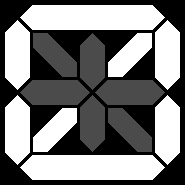0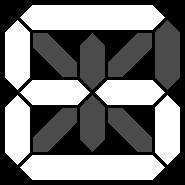6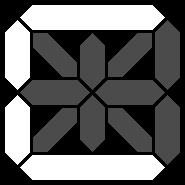C = 12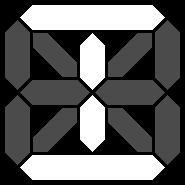I = 18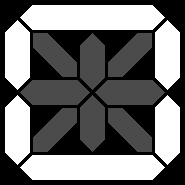O = 24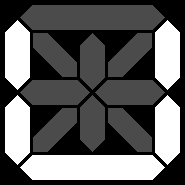U = 30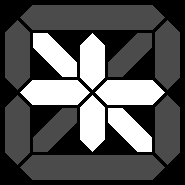-0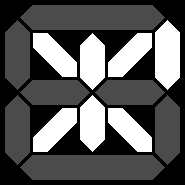-6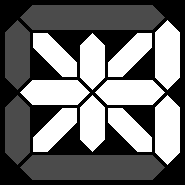-C = -12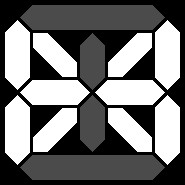-I = -18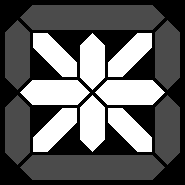-O = -24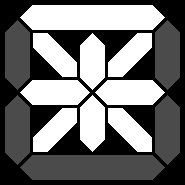-U = -30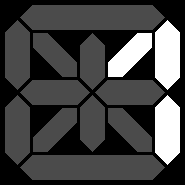1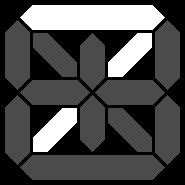7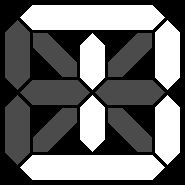D = 13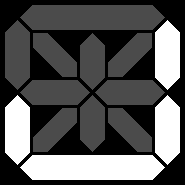J = 19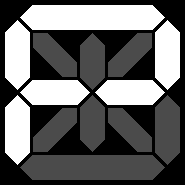P = 25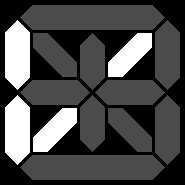V = 31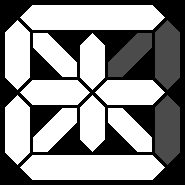-1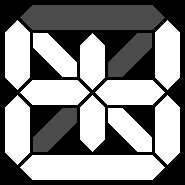-7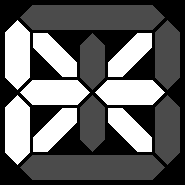-D = -13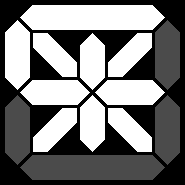-J = -19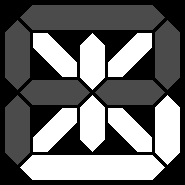-P = -25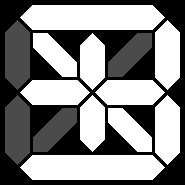-V = -31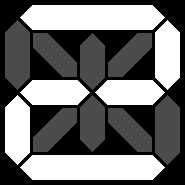2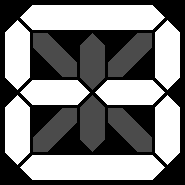8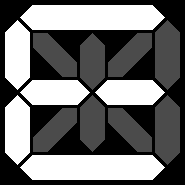E = 14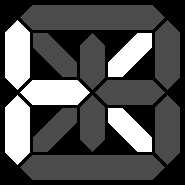K = 20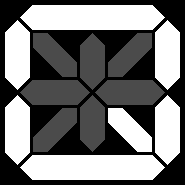Q = 26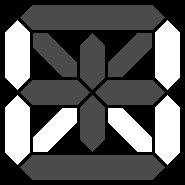W = 32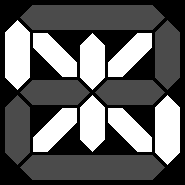-2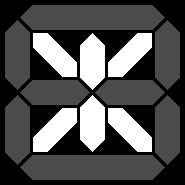-8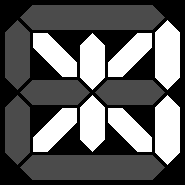-E = -14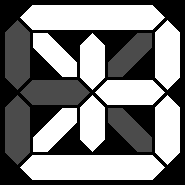-K = -20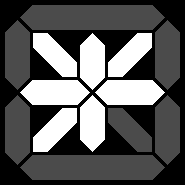-Q = -26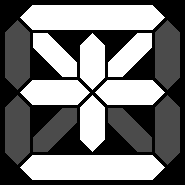-W = -32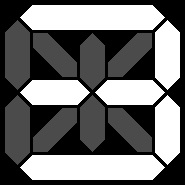3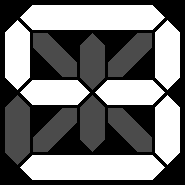9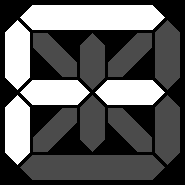F = 15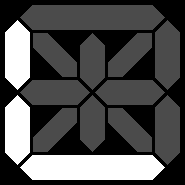L = 21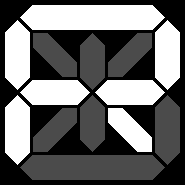R = 27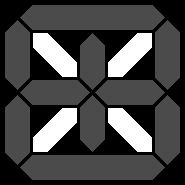X = 33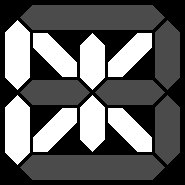-3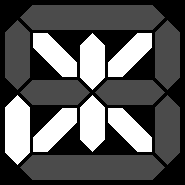-9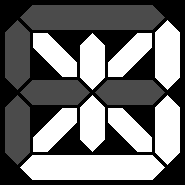-F = -15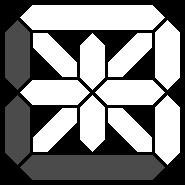-L = -21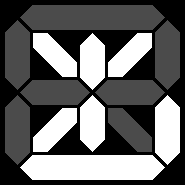-R = -27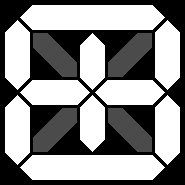-X = -33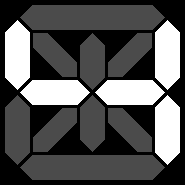4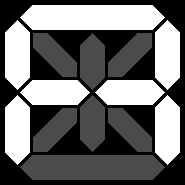A = 10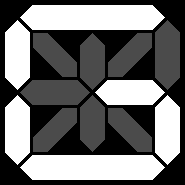G = 16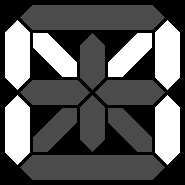M = 22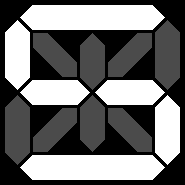S = 28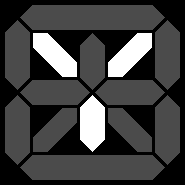Y = 34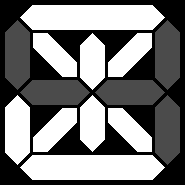-4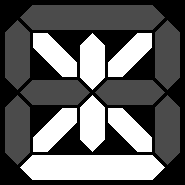-A = -10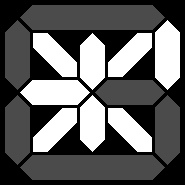-G = -16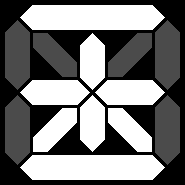-M = -22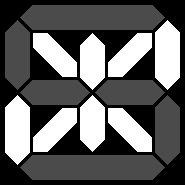-S = -28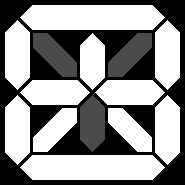-Y = -34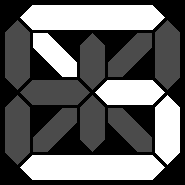5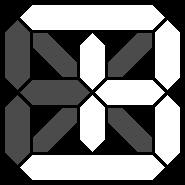B = 11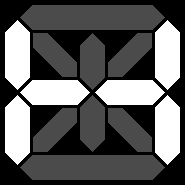H = 17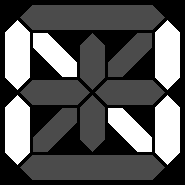N = 23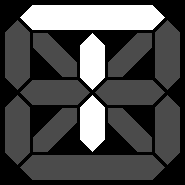T = 29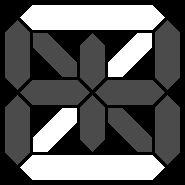Z = 35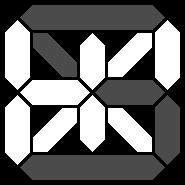-5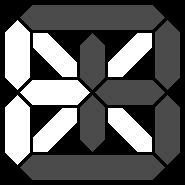-B = -11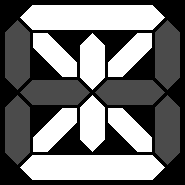-H = -17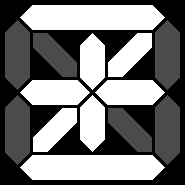-N = -23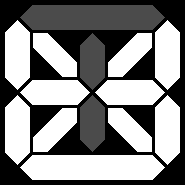-T = -29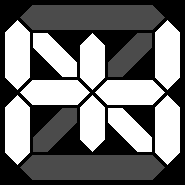-Z = -35